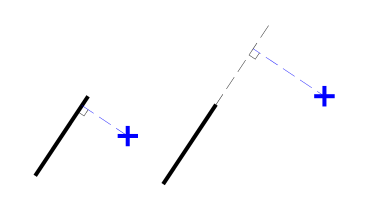# Distance of a Point from a Line (from CGA FAQ)

The distance of a point from a line is the length of a perpendicular drawn from the point to the line. Here are the possibilities:

• Perpendicular meets on the line segment
• Perpendicular meets on a forward/backward extension of the line segmentThe details of the solution can be found in the CGA FAQ linked above. A C++ implementation can be found here: http://code.google.com/p/curve-project/source/browse/trunk/lab/cgafaq/cgafaq.h The function cgafaq::distance_from_line takes the start and end points of the line and the point from which distance is to be calculated. r and s are output parameters calculated as described in the FAQ.

Note that if distance is not important and only a perpendicular needs to be generated then there is a simpler way to construct one.

Disclaimer: This post and the code provided are not endorsed by any of the contributors to the CGA FAQ.